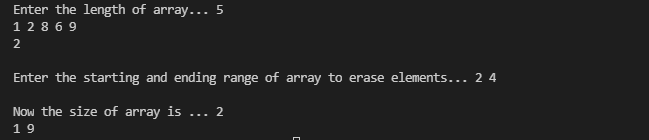The first line of the input contains an integer length. The next line contains length space-separated

integers(1-based index). The third line contains a single integer number, denoting the position of

an element that should be removed from the vector. The fourth line contains two integers a

and b denoting the range that should be erased from the vector inclusive of a and exclusive of b.

Print the size of the vector in the first line and the elements of the vector after the two erase operations

in the second line separated by space.

Sample Input:

Enter the length of array... 5

1 2 8 6 9

Enter the starting and ending range of the array to erase elements...

2 4

Sample Output:

Now the size of the array is ... 2

1 9

#include <cmath>
#include <cstdio>
#include <vector>
#include <iostream>
#include <algorithm>
using namespace std;

int main()
{
/* Enter your code here. Read input from STDIN. Print output to STDOUT */
int len;
int number;
int a, b;
cout << "Enter the length of array... ";
cin >> len;
vector<int> v1(len);
for (int i = 0; i < len; i++)
{
cin >> v1[i];
cin.ignore();
}
cin >> len;
v1.erase(v1.begin() + (len - 1));
cout << "\nEnter the starting and ending range of array to erase elements... ";
cin >> a >> b;
v1.erase(v1.begin() + (a - 1), v1.begin() + (b - 1));
cout << "\nNow the size of array is ... ";
cout << v1.size() << endl;
for (int i : v1)
{
cout << i << " ";
}

return 0;
}cpp programming exercise with solution